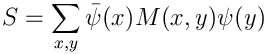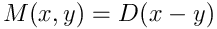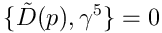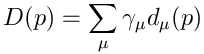## Monday, December 05, 2005

### The Nielsen-Ninomiya theorem

In recent posts in this series, we have been looking at naive, Wilson and staggered fermions. One of the things we have seen is how difficult it is to get rid of the doubler fermions; staggering did a good job at this, but still retained some of the doublers with all the problems they bring, while the Wilson term got rid of the doublers, but only at the expense of spoiling chiral symmetry, which brought on even worse problems. Why should the discretisation of fermions be so hard?

The answer lies in a theorem about lattice fermions, the celebrated Nielsen-Ninomiya no-go theorem, which states that it is impossible to have a chirally invariant, doubler-free, local, translation invariant, real bilinear fermion action on the lattice. The theorem comes from topological arguments: A real bilinear fermion action can be written aswith hermitean $$M$$. Translation invariance means thatand locality requires that the Fourier transform $$\tilde{D}(p)$$ of $$D(z)$$ be a regular function of p throughout the Brillouin zone. Chiral symmetryrequires thatSince the Brillouin zone has the topology of a 4-torus, we thus have a vector field $$d_\mu$$ on the torus. Now it is possible to assign an "index" of +1 or -1 to every zero of this vector field, and the Hopf-Poincare index theorem states that the sum over the indices of the zeros of a vector field on a manifold is equal to the Euler characteristic of the manifold. The Euler characteristic of any n-torus is zero, and therefore the zeros of $$d_\mu$$ must come in pairs of opposite index, which is precisely the origin of the doublers.

OK, so what does all this mathematics mean? Well, prima facie it seems to leave us with the choice between chiral symmetry and freedom from doublers (since locality, translation invariance and hermiticity are too important to abandon). There is, however, a clever way around this, which will be the topic of our next post.